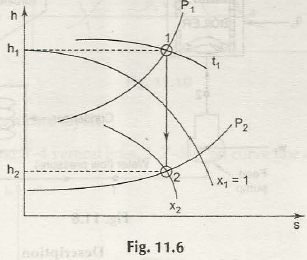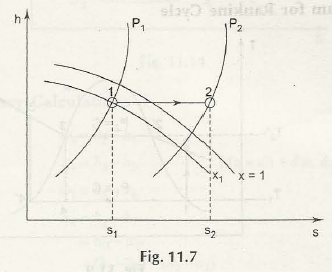In Mollier diagram the following lines are given

Constant P- lines

Constant v- lines

Constant t- lines

Constant x- lines

Constant h– lines (horizontal)

Constant s – lines (vertical)The process of isentropic where s=c

In Point 2 we take s= constant and then we go on to draw a vertical line from 1 to intersect P which is outlined at 2. We can observe it as an example that the steam is wet at 2 where dryness fraction of x2 (< x1) is seen. In this regard the enthalpy drop h1- h2 can be read out from the chart.The process of throttling where he= const

At point one the intersection of P1 and P2 is observed. Then in point 2 you need to go on and draw a horizontal line where P2at P meets. From the diagram it is seen that point 2 is superheated.

Links of Previous Main Topic:-

Links of Next Mechanical Engineering Topics:-

### Submit Your Assignment### Customer Reviews

My Homework Help
Rated 5.0 out of 5 based on 510 customer reviews at